# The MODEL Procedure

### Autoregressive Moving-Average Error Processes

Subsections:

Autoregressive moving-average error processes (ARMA errors) and other models that involve lags of error terms can be estimated by using FIT statements and simulated or forecast by using SOLVE statements. ARMA models for the error process are often used for models with autocorrelated residuals. The %AR macro can be used to specify models with autoregressive error processes. The %MA macro can be used to specify models with moving-average error processes.

#### Autoregressive Errors

A model with first-order autoregressive errors, AR(1), has the form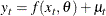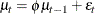while an AR(2) error process has the form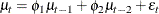and so forth for higher-order processes. Note that the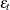’s are independent and identically distributed and have an expected value of 0.

An example of a model with an AR(2) component is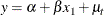You would write this model as

proc model data=in;
parms a b p1 p2;
y = a + b * x1 + p1 * zlag1(y - (a + b * x1)) +
p2 * zlag2(y - (a + b * x1));
fit y;
run;


or equivalently using the %AR macro as

proc model data=in;
parms a b;
y = a + b * x1;
%ar( y, 2 );
fit y;
run;


#### Moving-Average Models

A model with first-order moving-average errors, MA(1), has the form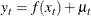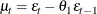whereis identically and independently distributed with mean zero. An MA(2) error process has the form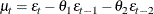and so forth for higher-order processes.

For example, you can write a simple linear regression model with MA(2) moving-average errors as

   proc model data=inma2;
parms a b ma1 ma2;
y = a + b * x + ma1 * zlag1( resid.y ) +
ma2 * zlag2( resid.y );
fit;
run;


where MA1 and MA2 are the moving-average parameters.

Note that RESID.Y is automatically defined by PROC MODEL as

   pred.y = a + b * x + ma1 * zlag1( resid.y ) +
ma2 * zlag2( resid.y );
resid.y = pred.y - actual.y;


Note that RESID.Y is negative of.

The ZLAG function must be used for MA models to truncate the recursion of the lags. This ensures that the lagged errors start at zero in the lag-priming phase and do not propagate missing values when lag-priming period variables are missing, and it ensures that the future errors are zero rather than missing during simulation or forecasting. For details about the lag functions, see the section Lag Logic.

This model written using the %MA macro is as follows:

   proc model data=inma2;
parms a b;
y =  a + b * x;
%ma(y, 2);
fit;
run;


#### General Form for ARMA Models

The general ARMA(p,q ) process has the following form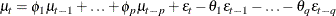An ARMA(p,q ) model can be specified as follows:

   yhat =  ... compute structural predicted value here ... ;
yarma = ar1 * zlag1( y - yhat ) + ...  /* ar part */
+ ar(p) * zlag(p)( y - yhat )
+ ma1 * zlag1( resid.y )   + ...  /* ma part */
+ ma(q) * zlag(q)( resid.y );
y = yhat + yarma;


where ARi and MAj represent the autoregressive and moving-average parameters for the various lags. You can use any names you want for these variables, and there are many equivalent ways that the specification could be written.

Vector ARMA processes can also be estimated with PROC MODEL. For example, a two-variable AR(1) process for the errors of the two endogenous variables Y1 and Y2 can be specified as follows:

   y1hat =  ... compute structural predicted value here ... ;

y1    = y1hat + ar1_1 * zlag1( y1 - y1hat )   /* ar part y1,y1 */
+ ar1_2 * zlag1( y2 - y2hat );  /* ar part y1,y2 */

y21hat =  ... compute structural predicted value here ... ;

y2     = y2hat + ar2_2 * zlag1( y2 - y2hat )   /* ar part y2,y2 */
+ ar2_1 * zlag1( y1 - y1hat );  /* ar part y2,y1 */


#### Convergence Problems with ARMA Models

ARMA models can be difficult to estimate. If the parameter estimates are not within the appropriate range, a moving-average model’s residual terms grow exponentially. The calculated residuals for later observations can be very large or can overflow. This can happen either because improper starting values were used or because the iterations moved away from reasonable values.

Care should be used in choosing starting values for ARMA parameters. Starting values of 0.001 for ARMA parameters usually work if the model fits the data well and the problem is well-conditioned. Note that an MA model can often be approximated by a high-order AR model, and vice versa. This can result in high collinearity in mixed ARMA models, which in turn can cause serious ill-conditioning in the calculations and instability of the parameter estimates.

If you have convergence problems while estimating a model with ARMA error processes, try to estimate in steps. First, use a FIT statement to estimate only the structural parameters with the ARMA parameters held to zero (or to reasonable prior estimates if available). Next, use another FIT statement to estimate the ARMA parameters only, using the structural parameter values from the first run. Since the values of the structural parameters are likely to be close to their final estimates, the ARMA parameter estimates might now converge. Finally, use another FIT statement to produce simultaneous estimates of all the parameters. Since the initial values of the parameters are now likely to be quite close to their final joint estimates, the estimates should converge quickly if the model is appropriate for the data.

#### AR Initial Conditions

The initial lags of the error terms of AR(p ) models can be modeled in different ways. The autoregressive error startup methods supported by SAS/ETS procedures are the following:

CLS

conditional least squares (ARIMA and MODEL procedures)

ULS

unconditional least squares (AUTOREG, ARIMA, and MODEL procedures)

ML

maximum likelihood (AUTOREG, ARIMA, and MODEL procedures)

YW

Yule-Walker (AUTOREG procedure only)

HL

Hildreth-Lu, which deletes the first p observations (MODEL procedure only)

See Chapter 8: The AUTOREG Procedure, for an explanation and discussion of the merits of various AR(p) startup methods.

The CLS, ULS, ML, and HL initializations can be performed by PROC MODEL. For AR(1) errors, these initializations can be produced as shown in Table 19.2. These methods are equivalent in large samples.

Table 19.2: Initializations Performed by PROC MODEL: AR(1) ERRORS

Method

Formula

conditional least squares

Y=YHAT+AR1*ZLAG1(Y-YHAT);

unconditional least squares

Y=YHAT+AR1*ZLAG1(Y-YHAT);

IF _OBS_=1 THEN

RESID.Y=SQRT(1-AR1**2)*RESID.Y;

maximum likelihood

Y=YHAT+AR1*ZLAG1(Y-YHAT);

W=(1-AR1**2)**(-1/(2*_NUSED_));

IF _OBS_=1 THEN W=W*SQRT(1-AR1**2);

RESID.Y=W*RESID.Y;

Hildreth-Lu

Y=YHAT+AR1*LAG1(Y-YHAT);

#### MA Initial Conditions

The initial lags of the error terms of MA(q ) models can also be modeled in different ways. The following moving-average error start-up paradigms are supported by the ARIMA and MODEL procedures:

ULS

unconditional least squares

CLS

conditional least squares

ML

maximum likelihood

The conditional least squares method of estimating moving-average error terms is not optimal because it ignores the start-up problem. This reduces the efficiency of the estimates, although they remain unbiased. The initial lagged residuals, extending before the start of the data, are assumed to be 0, their unconditional expected value. This introduces a difference between these residuals and the generalized least squares residuals for the moving-average covariance, which, unlike the autoregressive model, persists through the data set. Usually this difference converges quickly to 0, but for nearly noninvertible moving-average processes the convergence is quite slow. To minimize this problem, you should have plenty of data, and the moving-average parameter estimates should be well within the invertible range.

This problem can be corrected at the expense of writing a more complex program. Unconditional least squares estimates for the MA(1) process can be produced by specifying the model as follows:

   yhat =  ... compute structural predicted value here ... ;
if _obs_ = 1 then do;
h = sqrt( 1 + ma1 ** 2 );
y = yhat;
resid.y = ( y - yhat ) / h;
end;
else do;
g = ma1 / zlag1( h );
h = sqrt( 1 + ma1 ** 2 - g ** 2 );
y = yhat + g * zlag1( resid.y );
resid.y = ( ( y - yhat) - g * zlag1( resid.y ) ) / h;
end;


Moving-average errors can be difficult to estimate. You should consider using an AR(p ) approximation to the moving-average process. A moving-average process can usually be well-approximated by an autoregressive process if the data have not been smoothed or differenced.

#### The %AR Macro

The SAS macro %AR generates programming statements for PROC MODEL for autoregressive models. The %AR macro is part of SAS/ETS software, and no special options need to be set to use the macro. The autoregressive process can be applied to the structural equation errors or to the endogenous series themselves.

The %AR macro can be used for the following types of autoregression:

• univariate autoregression

• unrestricted vector autoregression

• restricted vector autoregression

#### Univariate Autoregression

To model the error term of an equation as an autoregressive process, use the following statement after the equation:

   %ar( varname, nlags )


For example, suppose that Y is a linear function of X1, X2, and an AR(2) error. You would write this model as follows:

proc model data=in;
parms a b c;
y = a + b * x1 + c * x2;
%ar( y, 2 )
fit y / list;
run;


The calls to %AR must come after all of the equations that the process applies to.

The preceding macro invocation, %AR(y,2), produces the statements shown in the LIST output in Figure 19.60.

Figure 19.60: LIST Option Output for an AR(2) Model

The MODEL Procedure

Listing of Compiled Program Code
Stmt Line:Col Statement as Parsed
1 2498:4 PRED.y = a + b * x1 + c * x2;
1 2498:4 RESID.y = PRED.y - ACTUAL.y;
1 2498:4 ERROR.y = PRED.y - y;
2 2499:14 _PRED__y = PRED.y;
3 2499:15 _OLD_PRED.y = PRED.y + y_l1 * ZLAG1( y - _PRED__y ) + y_l2 * ZLAG2( y - _PRED__y );
3 2499:15 PRED.y = _OLD_PRED.y;
3 2499:15 RESID.y = PRED.y - ACTUAL.y;
3 2499:15 ERROR.y = PRED.y - y;

The _PRED__ prefixed variables are temporary program variables used so that the lags of the residuals are the correct residuals and not the ones redefined by this equation. Note that this is equivalent to the statements explicitly written in the section General Form for ARMA Models.

You can also restrict the autoregressive parameters to zero at selected lags. For example, if you wanted autoregressive parameters at lags 1, 12, and 13, you can use the following statements:

proc model data=in;
parms a b c;
y = a + b * x1 + c * x2;
%ar( y, 13, , 1 12 13 )
fit y / list;
run;


These statements generate the output shown in Figure 19.61.

Figure 19.61: LIST Option Output for an AR Model with Lags at 1, 12, and 13

The MODEL Procedure

Listing of Compiled Program Code
Stmt Line:Col Statement as Parsed
1 2507:4 PRED.y = a + b * x1 + c * x2;
1 2507:4 RESID.y = PRED.y - ACTUAL.y;
1 2507:4 ERROR.y = PRED.y - y;
2 2508:14 _PRED__y = PRED.y;
3 2508:15 _OLD_PRED.y = PRED.y + y_l1 * ZLAG1( y - _PRED__y ) + y_l12 * ZLAG12( y - _PRED__y ) + y_l13 * ZLAG13( y - _PRED__y );
3 2508:15 PRED.y = _OLD_PRED.y;
3 2508:15 RESID.y = PRED.y - ACTUAL.y;
3 2508:15 ERROR.y = PRED.y - y;

There are variations on the conditional least squares method, depending on whether observations at the start of the series are used to "warm up" the AR process. By default, the %AR conditional least squares method uses all the observations and assumes zeros for the initial lags of autoregressive terms. By using the M= option, you can request that %AR use the unconditional least squares (ULS) or maximum-likelihood (ML) method instead. For example,

proc model data=in;
y = a + b * x1 + c * x2;
%ar( y, 2, m=uls )
fit y;
run;


Discussions of these methods is provided in the section AR Initial Conditions.

By using the M=CLSn option, you can request that the first n observations be used to compute estimates of the initial autoregressive lags. In this case, the analysis starts with observation n +1. For example:

proc model data=in;
y = a + b * x1 + c * x2;
%ar( y, 2, m=cls2 )
fit y;
run;


You can use the %AR macro to apply an autoregressive model to the endogenous variable, instead of to the error term, by using the TYPE=V option. For example, if you want to add the five past lags of Y to the equation in the previous example, you could use %AR to generate the parameters and lags by using the following statements:

proc model data=in;
parms a b c;
y = a + b * x1 + c * x2;
%ar( y, 5, type=v )
fit y / list;
run;


The preceding statements generate the output shown in Figure 19.62.

Figure 19.62: LIST Option Output for an AR model of Y

The MODEL Procedure

Listing of Compiled Program Code
Stmt Line:Col Statement as Parsed
1 2530:4 PRED.y = a + b * x1 + c * x2;
1 2530:4 RESID.y = PRED.y - ACTUAL.y;
1 2530:4 ERROR.y = PRED.y - y;
2 2531:15 _OLD_PRED.y = PRED.y + y_l1 * ZLAG1( y ) + y_l2 * ZLAG2( y ) + y_l3 * ZLAG3( y ) + y_l4 * ZLAG4( y ) + y_l5 * ZLAG5( y );
2 2531:15 PRED.y = _OLD_PRED.y;
2 2531:15 RESID.y = PRED.y - ACTUAL.y;
2 2531:15 ERROR.y = PRED.y - y;

This model predicts Y as a linear combination of X1, X2, an intercept, and the values of Y in the most recent five periods.

#### Unrestricted Vector Autoregression

To model the error terms of a set of equations as a vector autoregressive process, use the following form of the %AR macro after the equations:

   %ar( process_name, nlags, variable_list )


The process_name value is any name that you supply for %AR to use in making names for the autoregressive parameters. You can use the %AR macro to model several different AR processes for different sets of equations by using different process names for each set. The process name ensures that the variable names used are unique. Use a short process_name value for the process if parameter estimates are to be written to an output data set. The %AR macro tries to construct parameter names less than or equal to eight characters, but this is limited by the length of process_name, which is used as a prefix for the AR parameter names.

The variable_list value is the list of endogenous variables for the equations.

For example, suppose that errors for equations Y1, Y2, and Y3 are generated by a second-order vector autoregressive process. You can use the following statements:

   proc model data=in;
y1 = ... equation for y1 ...;
y2 = ... equation for y2 ...;
y3 = ... equation for y3 ...;
%ar( name, 2, y1 y2 y3 )
fit y1 y2 y3;
run;


which generate the following for Y1 and similar code for Y2 and Y3:

   y1 = pred.y1 + name1_1_1*zlag1(y1-name_y1) +
name1_1_2*zlag1(y2-name_y2) +
name1_1_3*zlag1(y3-name_y3) +
name2_1_1*zlag2(y1-name_y1) +
name2_1_2*zlag2(y2-name_y2) +
name2_1_3*zlag2(y3-name_y3) ;


Only the conditional least squares (M=CLS or M=CLSn ) method can be used for vector processes.

You can also use the same form with restrictions that the coefficient matrix be 0 at selected lags. For example, the following statements apply a third-order vector process to the equation errors with all the coefficients at lag 2 restricted to 0 and with the coefficients at lags 1 and 3 unrestricted:

   proc model data=in;
y1 = ... equation for y1 ...;
y2 = ... equation for y2 ...;
y3 = ... equation for y3 ...;
%ar( name, 3, y1 y2 y3, 1 3 )
fit y1 y2 y3;


You can model the three series Y1–Y3 as a vector autoregressive process in the variables instead of in the errors by using the TYPE=V option. If you want to model Y1–Y3 as a function of past values of Y1–Y3 and some exogenous variables or constants, you can use %AR to generate the statements for the lag terms. Write an equation for each variable for the nonautoregressive part of the model, and then call %AR with the TYPE=V option. For example,

proc model data=in;
parms a1-a3 b1-b3;
y1 = a1 + b1 * x;
y2 = a2 + b2 * x;
y3 = a3 + b3 * x;
%ar( name, 2, y1 y2 y3, type=v )
fit y1 y2 y3;
run;


The nonautoregressive part of the model can be a function of exogenous variables, or it can be intercept parameters. If there are no exogenous components to the vector autoregression model, including no intercepts, then assign zero to each of the variables. There must be an assignment to each of the variables before %AR is called.

proc model data=in;
y1=0;
y2=0;
y3=0;
%ar( name, 2, y1 y2 y3, type=v )
fit y1 y2 y3;
run;


This example models the vector Y=(Y1 Y2 Y3)as a linear function only of its value in the previous two periods and a white noise error vector. The model has 18=(33 + 33) parameters.

#### Syntax of the %AR Macro

There are two cases of the syntax of the %AR macro. When restrictions on a vector AR process are not needed, the syntax of the %AR macro has the general form

• %AR ( name , nlag <,endolist <, laglist >> <,M= method > <,TYPE= V> );

where

name

specifies a prefix for %AR to use in constructing names of variables needed to define the AR process. If the endolist is not specified, the endogenous list defaults to name, which must be the name of the equation to which the AR error process is to be applied. The name value cannot exceed 32 characters.

nlag

is the order of the AR process.

endolist

specifies the list of equations to which the AR process is to be applied. If more than one name is given, an unrestricted vector process is created with the structural residuals of all the equations included as regressors in each of the equations. If not specified, endolist defaults to name.

laglist

specifies the list of lags at which the AR terms are to be added. The coefficients of the terms at lags not listed are set to 0. All of the listed lags must be less than or equal to nlag, and there must be no duplicates. If not specified, the laglist defaults to all lags 1 through nlag.

M=method

specifies the estimation method to implement. Valid values of M= are CLS (conditional least squares estimates), ULS (unconditional least squares estimates), and ML (maximum likelihood estimates). M=CLS is the default. Only M=CLS is allowed when more than one equation is specified. The ULS and ML methods are not supported for vector AR models by %AR.

TYPE=V

specifies that the AR process is to be applied to the endogenous variables themselves instead of to the structural residuals of the equations.

#### Restricted Vector Autoregression

You can control which parameters are included in the process, restricting to 0 those parameters that you do not include. First, use %AR with the DEFER option to declare the variable list and define the dimension of the process. Then, use additional %AR calls to generate terms for selected equations with selected variables at selected lags. For example,

   proc model data=d;
y1 = ... equation for y1 ...;
y2 = ... equation for y2 ...;
y3 = ... equation for y3 ...;
%ar( name, 2, y1 y2 y3, defer )
%ar( name, y1, y1 y2 )
%ar( name, y2 y3, , 1 )
fit y1 y2 y3;
run;


The error equations produced are as follows:

   y1 = pred.y1 + name1_1_1*zlag1(y1-name_y1) +
name1_1_2*zlag1(y2-name_y2) + name2_1_1*zlag2(y1-name_y1) +
name2_1_2*zlag2(y2-name_y2) ;
y2 = pred.y2 + name1_2_1*zlag1(y1-name_y1) +
name1_2_2*zlag1(y2-name_y2) + name1_2_3*zlag1(y3-name_y3) ;
y3 = pred.y3 + name1_3_1*zlag1(y1-name_y1) +
name1_3_2*zlag1(y2-name_y2) + name1_3_3*zlag1(y3-name_y3) ;


This model states that the errors for Y1 depend on the errors of both Y1 and Y2 (but not Y3) at both lags 1 and 2, and that the errors for Y2 and Y3 depend on the previous errors for all three variables, but only at lag 1.

#### %AR Macro Syntax for Restricted Vector AR

An alternative use of %AR is allowed to impose restrictions on a vector AR process by calling %AR several times to specify different AR terms and lags for different equations.

The first call has the general form

• %AR( name, nlag, endolist , DEFER );

where

name

specifies a prefix for %AR to use in constructing names of variables needed to define the vector AR process.

nlag

specifies the order of the AR process.

endolist

specifies the list of equations to which the AR process is to be applied.

DEFER

specifies that %AR is not to generate the AR process but is to wait for further information specified in later %AR calls for the same name value.

The subsequent calls have the general form

   %AR( name, eqlist, varlist, laglist,TYPE= )


where

name

is the same as in the first call.

eqlist

specifies the list of equations to which the specifications in this %AR call are to be applied. Only names specified in the endolist value of the first call for the name value can appear in the list of equations in eqlist.

varlist

specifies the list of equations whose lagged structural residuals are to be included as regressors in the equations in eqlist. Only names in the endolist of the first call for the name value can appear in varlist. If not specified, varlist defaults to endolist.

laglist

specifies the list of lags at which the AR terms are to be added. The coefficients of the terms at lags not listed are set to 0. All of the listed lags must be less than or equal to the value of nlag, and there must be no duplicates. If not specified, laglist defaults to all lags 1 through nlag.

#### The %MA Macro

The SAS macro %MA generates programming statements for PROC MODEL for moving-average models. The %MA macro is part of SAS/ETS software, and no special options are needed to use the macro. The moving-average error process can be applied to the structural equation errors. The syntax of the %MA macro is the same as the %AR macro except there is no TYPE= argument.

When you are using the %MA and %AR macros combined, the %MA macro must follow the %AR macro. The following SAS/IML statements produce an ARMA(1, (1 3)) error process and save it in the data set MADAT2.

proc iml;
phi = { 1 .2 };
theta = { 1 .3 0 .5 };
y = armasim( phi, theta, 0, .1, 200, 32565 );
create madat2 from y[colname='y'];
append from y;
quit;


The following PROC MODEL statements are used to estimate the parameters of this model by using maximum likelihood error structure:

title 'Maximum Likelihood ARMA(1, (1 3))';
proc model data=madat2;
y=0;
%ar( y, 1, ,  M=ml )
%ma( y, 3, , 1 3,  M=ml )  /* %MA always after %AR */
fit y;
run;
title;


The estimates of the parameters produced by this run are shown in Figure 19.63.

Figure 19.63: Estimates from an ARMA(1, (1 3)) Process

 Maximum Likelihood ARMA(1, (1 3))

The MODEL Procedure

Nonlinear OLS Summary of Residual Errors
Equation DF Model DF Error SSE MSE Root MSE R-Square Adj R-Sq
y 3 197 2.6383 0.0134 0.1157 -0.0067 -0.0169
RESID.y   197 1.9957 0.0101 0.1007

Nonlinear OLS Parameter Estimates
Parameter Estimate Approx Std Err t Value Approx
Pr > |t|
Label
y_l1 -0.10067 0.1187 -0.85 0.3973 AR(y) y lag1 parameter
y_m1 -0.1934 0.0939 -2.06 0.0408 MA(y) y lag1 parameter
y_m3 -0.59384 0.0601 -9.88 <.0001 MA(y) y lag3 parameter

#### Syntax of the %MA Macro

There are two cases of the syntax for the %MA macro. When restrictions on a vector MA process are not needed, the syntax of the %MA macro has the general form

• %MA ( name , nlag <, endolist <, laglist >> <,M= method > );

where

name

specifies a prefix for %MA to use in constructing names of variables needed to define the MA process and is the default endolist.

nlag

is the order of the MA process.

endolist

specifies the equations to which the MA process is to be applied. If more than one name is given, CLS estimation is used for the vector process.

laglist

specifies the lags at which the MA terms are to be added. All of the listed lags must be less than or equal to nlag, and there must be no duplicates. If not specified, the laglist defaults to all lags 1 through nlag.

M=method

specifies the estimation method to implement. Valid values of M= are CLS (conditional least squares estimates), ULS (unconditional least squares estimates), and ML (maximum likelihood estimates). M=CLS is the default. Only M=CLS is allowed when more than one equation is specified in the endolist.

#### %MA Macro Syntax for Restricted Vector Moving-Average

An alternative use of %MA is allowed to impose restrictions on a vector MA process by calling %MA several times to specify different MA terms and lags for different equations.

The first call has the general form

• %MA( name , nlag , endolist , DEFER );

where

name

specifies a prefix for %MA to use in constructing names of variables needed to define the vector MA process.

nlag

specifies the order of the MA process.

endolist

specifies the list of equations to which the MA process is to be applied.

DEFER

specifies that %MA is not to generate the MA process but is to wait for further information specified in later %MA calls for the same name value.

The subsequent calls have the general form

   %MA( name, eqlist, varlist, laglist )


where

name

is the same as in the first call.

eqlist

specifies the list of equations to which the specifications in this %MA call are to be applied.

varlist

specifies the list of equations whose lagged structural residuals are to be included as regressors in the equations in eqlist.

laglist

specifies the list of lags at which the MA terms are to be added.